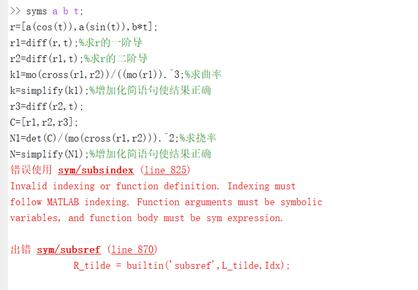m0_54185461 2021-11-30 15:15 采纳率: 100%

# 程序出现的问题，不知道如何补救。

``````syms a b t;
r=[a(cos(t)),a(sin(t)),b*t];
r1=diff(r,t);%求r的一阶导
r2=diff(r1,t);%求r的二阶导
k1=mo(cross(r1,r2))/((mo(r1)).^3;%求曲率
k=simplify(k1);%增加化简语句使结果正确
r3=diff(r2,t);
C=[r1,r2,r3];
N1=det(C)/(mo(cross(r1,r2))).^2;%求挠率
N=simplify(N1);%增加化简语句使结果正确

``````• 写回答

#### 悬赏问题

• ¥20 统信linux360企业浏览器 如何配置扩展程序？（图形界面我知道）
• ¥100 c/c++实现DoubleArrayTrie
• ¥30 关于#网络安全#的问题：非对称加密验证
• ¥20 关于线性代数里施密特正交化和QR分解的疑问
• ¥15 matlab超类包含解析错误
• ¥15 python拖拽文件问题